### Jan 12, 2015

I have been building images in Go and I would like to share how to build a chessboard image pattern with some modulo operations. In my case I am building a `6x6` grid of size `240px`. That means each square is a `40px` square.

I would like to build an image where one square (40x40) is black the next one white and so on. Also the colors alternate between each row.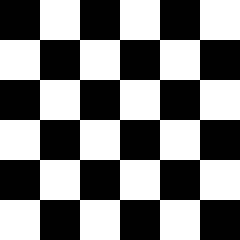#### Looping through the image:

To be able to make this chessboard we first need to loop through the X's and Y's of the image. Here is how you would create a black image by coloring each "pixel" in black.

`func drawBlack(m *image.RGBA, color1 color.RGBA) {    size := m.Bounds().Size()    for x := 0; x < size.X; x++ {        for y := 0; y < size.Y; y++ {            m.Set(x, y, color1)        }    }}`

#### Saving the image:

This is how you could save the image you built:

`func main() {    m := image.NewRGBA(image.Rect(0, 0, 240, 240))    black := color.RGBA{uint8(0), uint8(0), 0, 255}        drawBlack(m, black)        if img, err := os.Create("black.jpg"); err != nil {        log.Println("unable to create black.jpg: %v", err)    } else {        jpeg.Encode(img, m, nil)        defer img.Close()    }}`

#### modulo of ...:

The first thing that came to mind my mind was that I should use modulo of something..
We have a 2 level loop through X's and Y's. Let's try to build an image that draws black if `x%2 == 0` and draws white otherwise.

`func drawModuloOnX(m *image.RGBA, color1, color2 color.RGBA) {    size := m.Bounds().Size()    for x := 0; x < size.X; x++ {        for y := 0; y < size.Y; y++ {            if x%2 == 0 {                m.Set(x, y, color1)            } else {                m.Set(x, y, color2)            }        }    }}`
This is the image that we get: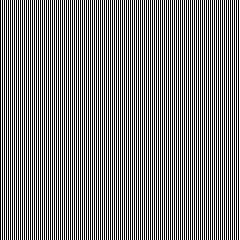If we do instead `y%2 == 0` we get the following: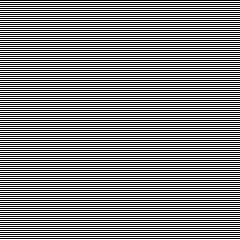How about `(x+y)%2 == 0`?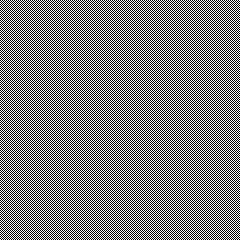Well, we are getting closer, but this is making a chessboard grid of 240x240 and what we want is a 6x6 chessboard grid. That means, to color a specific range/square with black and another range/square in white.

#### Indexes and ranges:

In order to color the image by a specific range let's take first a look at the first row of the image:

This is the index of that first row:

`0 1 2 ... 38 39 40 41 ... 78 79 80 81 ... 118 119 120 121 ... 158 159 160 161 ... 198 199 200 201 ... 238 239`
If we can get an array of the first row of the 6x6 grid to have 0 if the color is black and 1 if it is white, we are in good shape. We would like to build an array that transforms the indexes of this first row in the following:
`indexes:  0 1 2 ... 38 39 40 41 ... 78 79 80 81 ... 118 119 120 121 ... 158 159 160 161 ... 198 199 200 201 ... 238 239transform:0 0 0 ... 0  0  1  1  ... 1  1  0  0  ... 0   0   1   1   ... 1   1   0   0       0   0   1   1   ... 1   1`
To do this we need to first divide the indexes by the size of a square in this case 40. We get the following pattern:
`indexes:  0 1 2 ... 38 39 40 41 ... 78 79 80 81 ... 118 119 120 121 ... 158 159 160 161 ... 198 199 200 201 ... 238 239 /40:     0 0 0 ... 0  0  1  1  ... 1  1  2  2  ... 2   2   3   3   ... 3   3   4   4   ... 4   4   5   5   ... 5   5`
This is close to what we want. If we use the modulo of 2 now on the result of the previous array we get:
`indexes:  0 1 2 ... 38 39 40 41 ... 78 79 80 81 ... 118 119 120 121 ... 158 159 160 161 ... 198 199 200 201 ... 238 239transform:0 0 0 ... 0  0  1  1  ... 1  1  0  0  ... 0   0   1   1   ... 1   1   0   0       0   0   1   1   ... 1   1`

This looks good, let's build the image!

`func draw(m *image.RGBA, color1, color2 color.RGBA) {    size := m.Bounds().Size()    quadrant := size.X / 6    for x := 0; x < size.X; x++ {        val := (x / quadrant) % 2        for y := 0; y < size.Y; y++ {            if val == 0 {                m.Set(x, y, color1)            } else {                m.Set(x, y, color2)            }        }    }}`
Notice that in our case `size = 240` and`quadrant = 40`.
Now if we create our image using the above algorithm we get the following: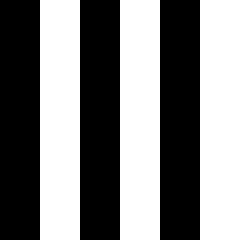Similarly if we now do `val = (y / quadrant) %2` we get the following: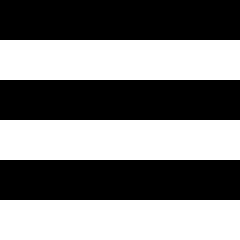#### Combining values:

As in section "modulo of ...", if we combine the vertical and horizontal values and compute a modulo:

`func drawGrid(m *image.RGBA, color1, color2 color.RGBA) {    size := m.Bounds().Size()    quad := size.X / 6    for x := 0; x < size.X; x++ {        val := (x / quad) % 2        for y := 0; y < size.Y; y++ {            val2 := (y / quad) % 2            if (val+val2)%2 == 0 {                m.Set(x, y, color1)            } else {                m.Set(x, y, color2)            }        }    }}`
We finally get the following \o/:I hope you found this interesting. Here is the final example also available in this gist

Some links that helped me making this: